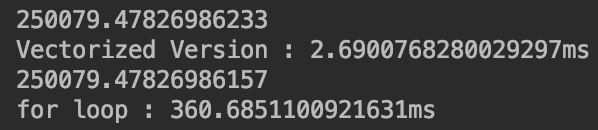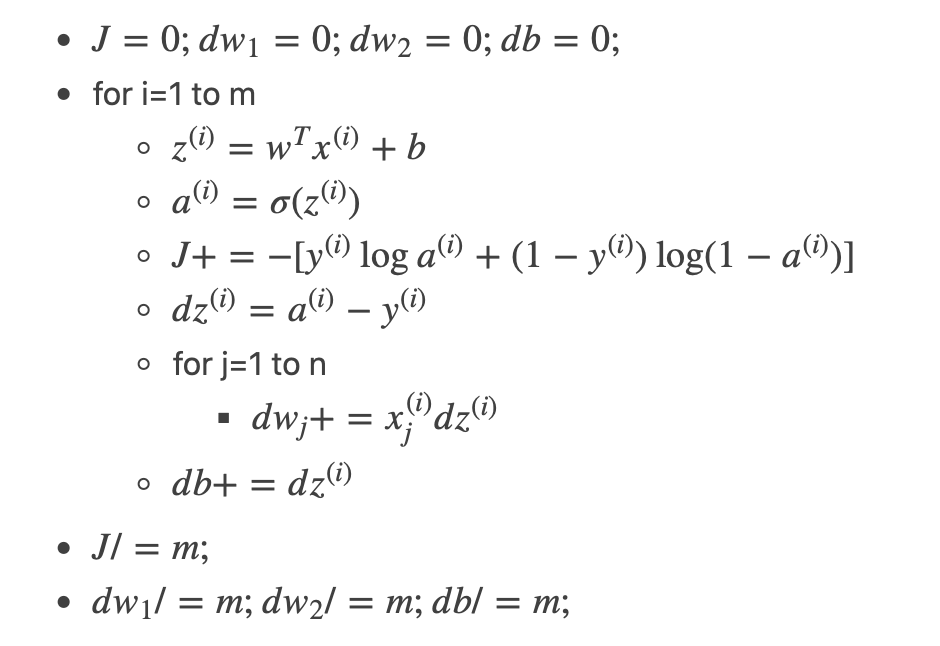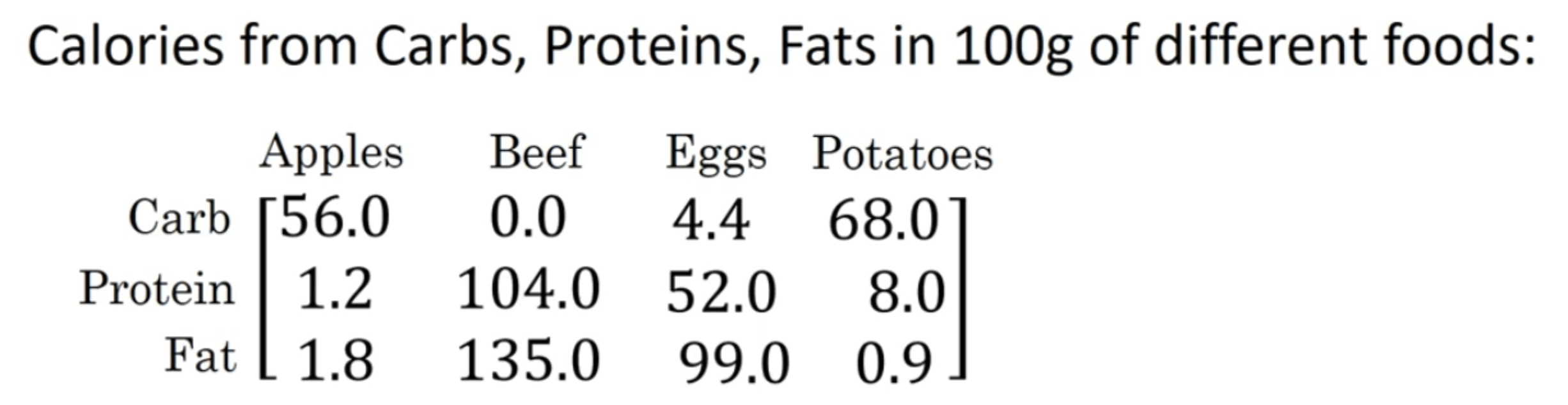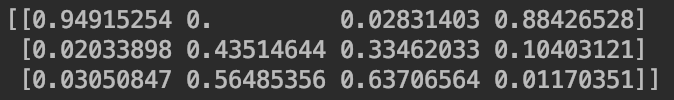# 【深度学习基础】第五课：向量化

Posted by x-jeff on November 22, 2019

【深度学习基础】系列博客为学习Coursera上吴恩达深度学习课程所做的课程笔记。

# 2.什么是向量化

$w=\begin{bmatrix} w_1 \\ \vdots \\ w_n \end{bmatrix};x=\begin{bmatrix} x_1 \\ \vdots \\ x_n \end{bmatrix}$

👉使用非向量化的方法：

1
2
3
4
z=0
for i in range n :
z+=w[i]*x[i]
z+=b


👉使用向量化的方法：

1
2
import numpy as np
z=np.dot(w,x)+b


1
2
3
4
5
6
7
8
9
10
11
12
13
14
15
16
a = np.random.rand(1000000) #构建1000000维的数组a
b = np.random.rand(1000000) #构建1000000维的数组b

tic = time.time()
c = np.dot(a,b) #向量化方法
toc = time.time()
print(c)
print("Vectorized Version : " + str(1000*(toc-tic)) + "ms")

c = 0
tic = time.time()
for i in range(1000000) :
c += a[i]*b[i] #使用for循环，非向量化方法
toc = time.time()
print(c)
print("for loop : " + str(1000*(toc-tic)) + "ms")## 2.1.更多的例子

👉假设向量v：

$v=\begin{bmatrix} v_1 \\ \vdots \\ v_n \end{bmatrix}$

$v=\begin{bmatrix} e^{v_1} \\ \vdots \\ e^{v_n} \end{bmatrix}$

1
2
3
u = np.zeros((n,1))
for i in range(n):
u[i]=math.exp(v[i])


1
2
import numpy as np
u = np.exp(v)


numpy中其他的类似用法：

1. u=np.log(v)
2. u=np.abs(v)
3. u=np.maximum(v,0) #返回v中每个元素和0之间的最大值
4. v**2 #计算v中每个元素的平方
5. 1/v #计算v中每个元素的倒数

# 3.使用向量化实现logistic回归梯度下降算法👉现在向量化第一个for循环：

$Z=[w_1,w_2,...,w_n] \begin{bmatrix} x^{(1)}_1 & x^{(2)}_1 & \cdots & x^{(m)}_1 \\ \vdots & \vdots & \vdots & \vdots \\ x^{(1)}_n & x^{(2)}_n & \cdots & x^{(m)}_n \\ \end{bmatrix} + [b,b,...,b]$

❗️在实际运算时，numpy会将实数b扩展为$[b,b,…,b]$，这个操作在python中叫做广播(broadcasting)

👉同样的，我们也可以将第二个for循环向量化：

## 4.1.例子一1
2
3
4
5
A = np.array([
[56.0, 0.0, 4.4, 68.0],
[1.2, 104.0, 52.0, 8.0],
[1.8, 135.0, 99.0, 0.9]
])


1
cal = A.sum(axis=0) #输出为：[ 59.  239.  155.4  76.9]


axis=0为各列求和；axis=1为各行求和。

1
percentage = A / cal.reshape(1,4)## 4.2.例子二

$\begin{bmatrix} 1 \\ 2 \\ 3 \\ 4 \\ \end{bmatrix} + 100$

$\begin{bmatrix} 1 \\ 2 \\ 3 \\ 4 \\ \end{bmatrix} + \begin{bmatrix} 100 \\ 100 \\ 100 \\ 100 \\ \end{bmatrix}$

$\begin{bmatrix} 1 & 2 & 3 \\ 4 & 5 & 6 \\ \end{bmatrix} + \begin{bmatrix} 100 & 200 & 300 \\ \end{bmatrix}$

$\begin{bmatrix} 1 & 2 & 3 \\ 4 & 5 & 6 \\ \end{bmatrix} + \begin{bmatrix} 100 & 200 & 300 \\ 100 & 200 & 300 \\ \end{bmatrix}$

$\begin{bmatrix} 1 & 2 & 3 \\ 4 & 5 & 6 \\ \end{bmatrix} + \begin{bmatrix} 100 \\ 200 \\ \end{bmatrix}$

$\begin{bmatrix} 1 & 2 & 3 \\ 4 & 5 & 6 \\ \end{bmatrix} + \begin{bmatrix} 100 & 100 & 100 \\ 200 & 200 & 200 \\ \end{bmatrix}$

# 5.关于numpy中的向量

numpy在赋予编码极大灵活性的同时也容易因使用不当而造成一些不易察觉、难以调试的bug。

1
2
import numpy as np
a = np.random.randn(5)


tips:

# 6.代码地址